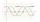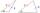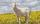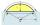# Equation + sine - math problems

#### Number of problems found: 18

• SinusDetermine the smallest integer p for which the equation 4 sin x = p has no solution.
• RightDetermine angles of the right triangle with the hypotenuse c and legs a, b, if: ?
• RhombusInternal angles of rhombus is in ratio 2:3. How many times is the shorter diagonal longer than side of rhombus?
• Two triangles SSATwo triangles can be formed with the given information. Use the Law of Sines to solve the triangles. A = 59°, a = 13, b = 14
• GoatMeadow is a circle with radius r = 19 m. How long must a rope to tie a goat to the pin on the perimeter of the meadow to allow goat eat half of meadow?
• Angle in RTDetermine the size of the smallest internal angle of a right triangle whose sides constitutes sizes consecutive members of arithmetic progressions.
• The hemisphereThe hemisphere container is filled with water. What is the radius of the container when 10 liters of water pour from it when tilted 30 degrees?
• Triangle ABC v2Area of the triangle is 12 cm square. Angle ACB = 30º , AC = (x + 2) cm, BC = x cm. Calculate the value of x.
• Right triangle eq2Find the lengths of the sides and the angles in the right triangle. Given area S = 210 and perimeter o = 70.
• Sphere in coneA sphere of radius 3 cm describes a cone with minimum volume. Determine cone dimensions.
• Two chordsFrom the point on the circle with a diameter of 8 cm, two identical chords are led, which form an angle of 60°. Calculate the length of these chords.
• Height of the arc - formulaCalculate the height of the arc if the length of the arc is 77 and chord length 40. Does exist a formula to solve this?
• CloudsApproximately at what height is the cloud we see under an angle of 26°10' and see the Sun at an angle of 29°15' and the shade of the cloud is 92 meters away from us?
• CloudsFrom two points A and B on the horizontal plane was observed forehead cloud above the two points under elevation angle 73°20' and 64°40'. Points A , B are separated by 2830 m. How high is the cloud?
• Trapezoid MOThe rectangular trapezoid ABCD with the right angle at point B, |AC| = 12, |CD| = 8, diagonals are perpendicular to each other. Calculate the perimeter and area of ​​the trapezoid.
• Viewing angleThe observer sees a straight fence 60 m long at a viewing angle of 30°. It is 102 m away from one end of the enclosure. How far is the observer from the other end of the enclosure?
• ObserverThe observer sees a straight fence 100 m long in 30° view angle. From one end of the fence is 102 m. How far is it from another end of the fence?
• Goat and circlesWhat is the radius of a circle centered on the other circle and the intersection of the two circles is equal to half the area of the first circle? This task is the mathematical expression of the role of agriculture. The farmer has circular land on which g

Do you have an interesting mathematical word problem that you can't solve it? Submit a math problem, and we can try to solve it.

We will send a solution to your e-mail address. Solved examples are also published here. Please enter the e-mail correctly and check whether you don't have a full mailbox.

Please do not submit problems from current active competitions such as Mathematical Olympiad, correspondence seminars etc...

Do you have a linear equation or system of equations and looking for its solution? Or do you have quadratic equation? Most natural application of trigonometry and trigonometric functions is a calculation of the triangles. Common and less common calculations of different types of triangles offers our triangle calculator. Word trigonometry comes from Greek and literally means triangle calculation. Equations Math problems. Sine - math word problems.